# Making A Single Heatmap

Author: Zuguang Gu ( z.gu@dkfz.de )

Date: 2017-04-24

A single heatmap is mostly used for a quick view of the data. It is a special case of a heatmap list which only contains one heatmap. Compare to available tools, ComplexHeatmap package provides a more flexible way to support visualization of a single heatmap. In following examples, we will demonstrate how to set parameters to visualize a single heatmap.

First let's load packages and generate a random matrix:

library(ComplexHeatmap)
library(circlize)

set.seed(123)

mat = cbind(rbind(matrix(rnorm(16, -1), 4), matrix(rnorm(32, 1), 8)),
rbind(matrix(rnorm(24, 1), 4), matrix(rnorm(48, -1), 8)))

# permute the rows and columns
mat = mat[sample(nrow(mat), nrow(mat)), sample(ncol(mat), ncol(mat))]

rownames(mat) = paste0("R", 1:12)
colnames(mat) = paste0("C", 1:10)


Plot the heatmap with default settings. The default style of the heatmap is quite the same as those generated by other similar heatmap functions.

Heatmap(mat)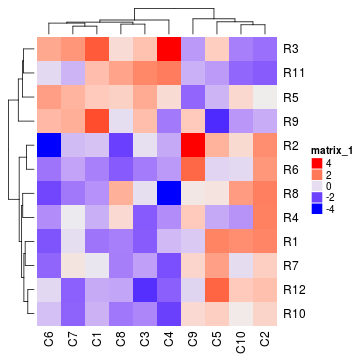## Colors

In most cases, the heatmap visualizes a matrix with continuous values. In this case, user should provide a color mapping function. A color mapping function should accept a vector of values and return a vector of corresponding colors. The colorRamp2() from the circlize package is helpful for generating such functions. The two arguments for colorRamp2() is a vector of breaks values and corresponding colors. Currently colorRamp2() linearly interpolates colors in every interval through LAB color space.

In following example, values between -3 and 3 are linearly interpolated to obtain corresponding colors, values larger than 3 are all mapped to red and values less than -3 are all mapped to green (so the color mapping function demonstrated here is robust to outliers).

mat2 = mat
mat2[1, 1] = 100000
Heatmap(mat2, col = colorRamp2(c(-3, 0, 3), c("green", "white", "red")),
cluster_rows = FALSE, cluster_columns = FALSE)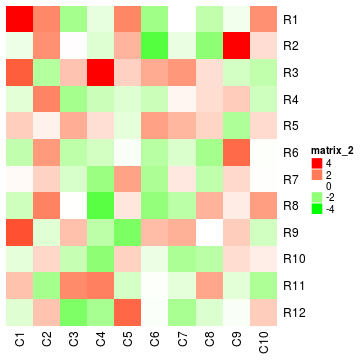If the matrix is continuous, you can also provide a vector of colors and colors will be interpolated according to the 'k'th quantile. But remember this method is not robust to outliers.

Heatmap(mat, col = rev(rainbow(10)))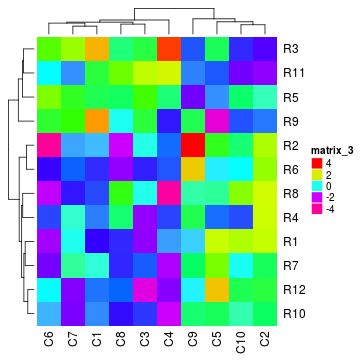If the matrix contains discrete values (either numeric or character), colors should be specified as a named vector to make it possible for the mapping from discrete values to colors. If there is no name for the color, the order of colors corresponds to the order of unique(mat).

discrete_mat = matrix(sample(1:4, 100, replace = TRUE), 10, 10)
colors = structure(circlize::rand_color(4), names = c("1", "2", "3", "4"))
Heatmap(discrete_mat, col = colors)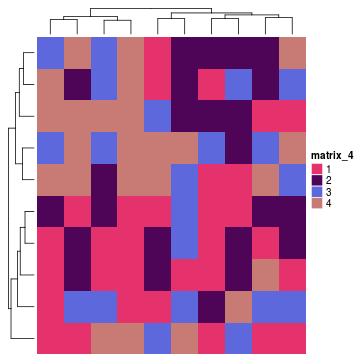Or a character matrix:

discrete_mat = matrix(sample(letters[1:4], 100, replace = TRUE), 10, 10)
colors = structure(circlize::rand_color(4), names = letters[1:4])
Heatmap(discrete_mat, col = colors)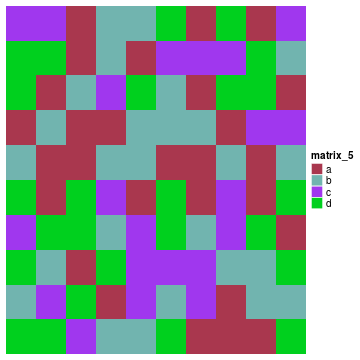As you see, for the numeric matrix (no matter it is continuous mapping or discrete mapping), by default clustering is applied on both dimensions while for character matrix, clustering is suppressed.

NA is allowed in the heatmap. You can control the color of NA by na_col argument. The matrix which contains NA can also be clustered by Heatmap() (since dist() accepts NA values) and clustering a matrix with NA values by “pearson”, “spearman” or “kendall” method gives warning messages.

mat_with_na = mat
mat_with_na[sample(c(TRUE, FALSE), nrow(mat)*ncol(mat), replace = TRUE, prob = c(1, 9))] = NA
Heatmap(mat_with_na, na_col = "orange", clustering_distance_rows = "pearson")

## Warning in get_dist(submat, distance): NA exists in the matrix, calculating distance by removing NA
## values.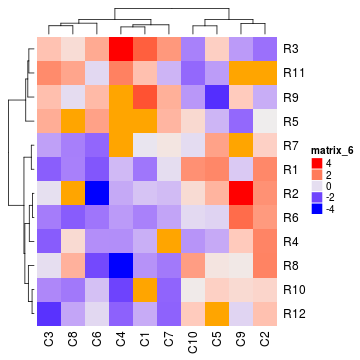Color space is important for interpolating colors. By default, colors are linearly interpolated in LAB color space, but you can select the color space in colorRamp2() function. Compare following two plots (+ operation on two heatmaps will be introduced in Making a list of heatmaps vignette):

f1 = colorRamp2(seq(min(mat), max(mat), length = 3), c("blue", "#EEEEEE", "red"))
f2 = colorRamp2(seq(min(mat), max(mat), length = 3), c("blue", "#EEEEEE", "red"), space = "RGB")
Heatmap(mat, col = f1, column_title = "LAB color space") +
Heatmap(mat, col = f2, column_title = "RGB color space")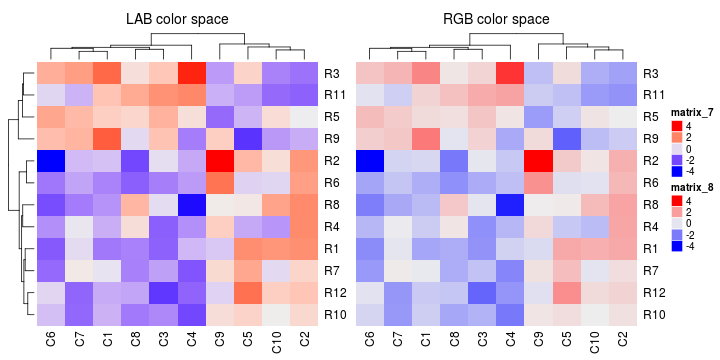On following figure, corresponding values change evenly on the folded axis, you can see how colors change under different color spaces (the plot is made by HilbertCurve package). Choosing a proper color space is a little bit subjective and it depends on specific data and color theme. Sometimes you need to try several color spaces to determine one which can best reveal potential structure of your data.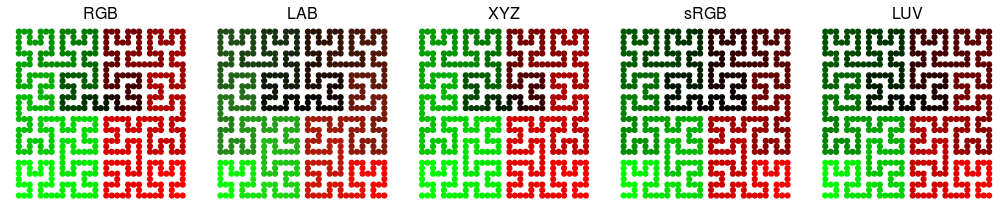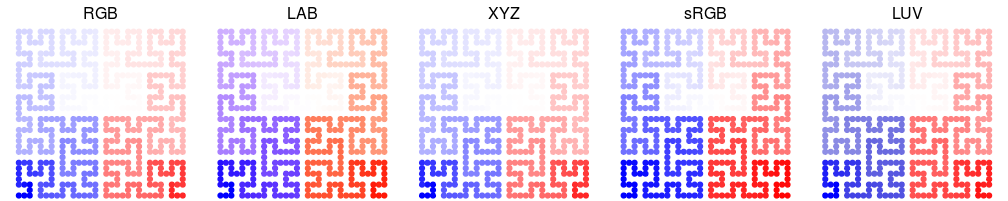## Titles

The name of the heatmap by default is used as the title of the heatmap legend. The name also plays as a unique id if you plot more than one heatmaps together. Later we can use this name to go to the corresponding heatmap to add more graphics (see Heatmap Decoration vignette).

Heatmap(mat, name = "foo")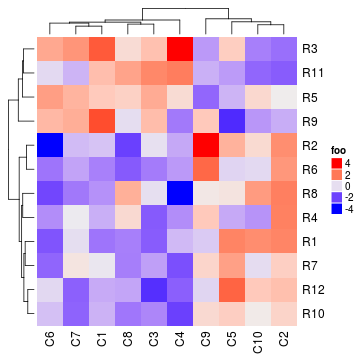The title of the heatmap legend can be modified by heatmap_legend_param (see Heatmap and Annotation Legends vignette for more control on the legend).

Heatmap(mat, heatmap_legend_param = list(title = "legend"))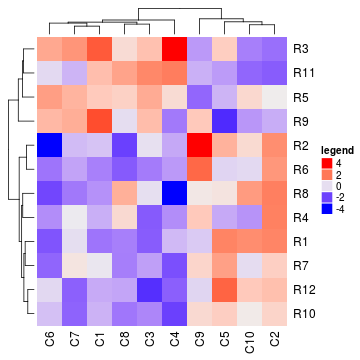You can set heatmap titles to be put either by the rows or by the columns. Note at a same time you can only put e.g. column title either on the top or at the bottom of the heatmap. The graphic parameters can be set by row_title_gp and column_title_gp respectively. Please remember you should use gpar() to specify graphic parameters.

Heatmap(mat, name = "foo", column_title = "I am a column title",
row_title = "I am a row title")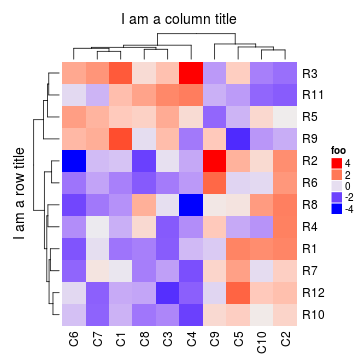Heatmap(mat, name = "foo", column_title = "I am a column title at the bottom",
column_title_side = "bottom")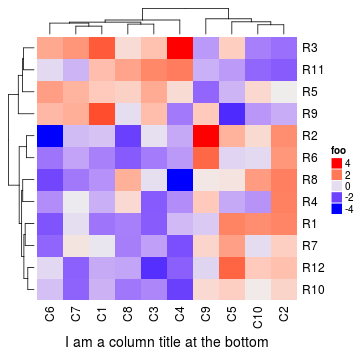Heatmap(mat, name = "foo", column_title = "I am a big column title",
column_title_gp = gpar(fontsize = 20, fontface = "bold"))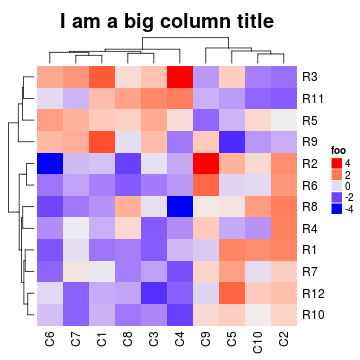Rotations for titles can be set by row_title_rot and column_title_rot, but only horizontal and vertical rotations are allowed.

Heatmap(mat, name = "foo", row_title = "row title", row_title_rot = 0)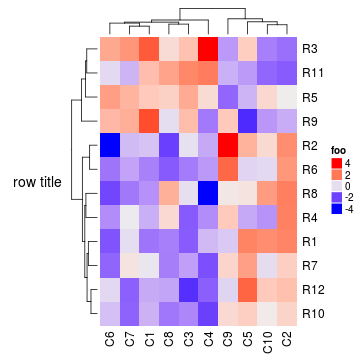## Clustering

Clustering may be the key feature of the heatmap visualization. In ComplexHeatmap package, clustering is supported with high flexibility. You can specify the clustering either by a pre-defined method (e.g. “eulidean” or “pearson”), or by a distance function, or by a object that already contains clustering, or directly by a clustering function. It is also possible to render your dendrograms with different colors and styles for different branches for better revealing structures of your data.

First there are general settings for the clustering, e.g. whether do or show dendrograms, side of the dendrograms and size of the dendrograms.

Heatmap(mat, name = "foo", cluster_rows = FALSE)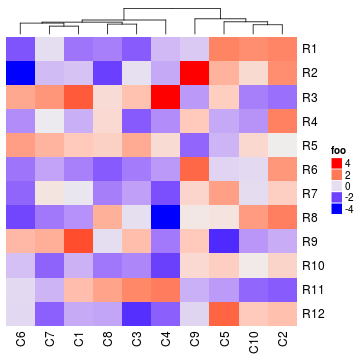Heatmap(mat, name = "foo", show_column_dend = FALSE)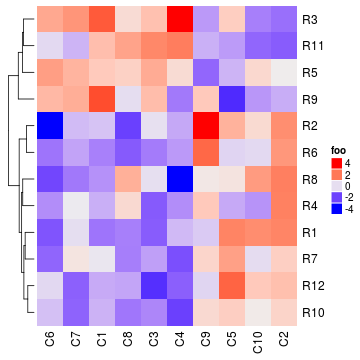Heatmap(mat, name = "foo", row_dend_side = "right")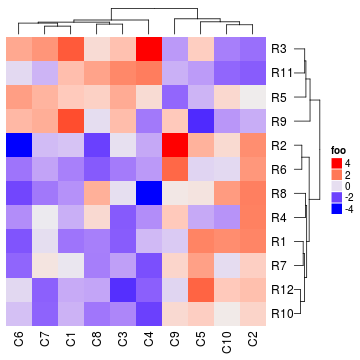Heatmap(mat, name = "foo", column_dend_height = unit(2, "cm"))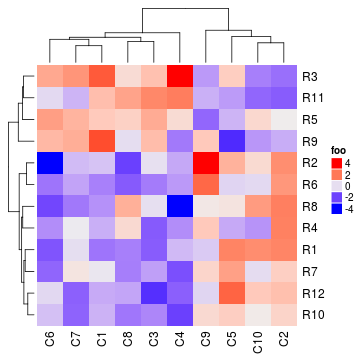There are three ways to specify distance metric for clustering:

• specify distance as a pre-defined option. The valid values are the supported methods in dist() function and within pearson, spearman and kendall. NA values are ignored for pre-defined clustering but with giving warnings (see example in Colors section).
• a self-defined function which calculates distance from a matrix. The function should only contain one argument. Please note for clustering on columns, the matrix will be transposed automatically.
• a self-defined function which calculates distance from two vectors. The function should only contain two arguments.
Heatmap(mat, name = "foo", clustering_distance_rows = "pearson")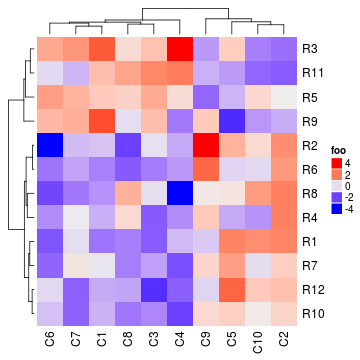Heatmap(mat, name = "foo", clustering_distance_rows = function(m) dist(m))Heatmap(mat, name = "foo", clustering_distance_rows = function(x, y) 1 - cor(x, y))Based on this feature, we can apply clustering which is robust to outliers based on the pair-wise distance.

mat_with_outliers = mat
for(i in  1:10) mat_with_outliers[i, i] = 1000
robust_dist = function(x, y) {
qx = quantile(x, c(0.1, 0.9))
qy = quantile(y, c(0.1, 0.9))
l = x > qx & x < qx & y > qy & y < qy
x = x[l]
y = y[l]
sqrt(sum((x - y)^2))
}
Heatmap(mat_with_outliers, name = "foo",
col = colorRamp2(c(-3, 0, 3), c("green", "white", "red")),
clustering_distance_rows = robust_dist,
clustering_distance_columns = robust_dist)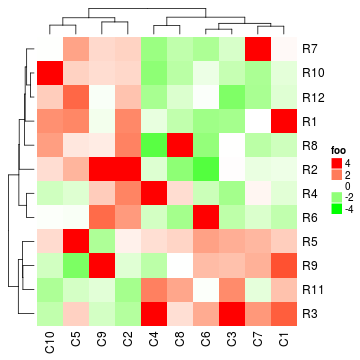If possible distance method provided, you can also cluster a character matrix. cell_fun argument will be explained in later section.

mat_letters = matrix(sample(letters[1:4], 100, replace = TRUE), 10)
# distance in th ASCII table
dist_letters = function(x, y) {
x = strtoi(charToRaw(paste(x, collapse = "")), base = 16)
y = strtoi(charToRaw(paste(y, collapse = "")), base = 16)
sqrt(sum((x - y)^2))
}
Heatmap(mat_letters, name = "foo", col = structure(2:5, names = letters[1:4]),
clustering_distance_rows = dist_letters, clustering_distance_columns = dist_letters,
cell_fun = function(j, i, x, y, w, h, col) {
grid.text(mat_letters[i, j], x, y)
})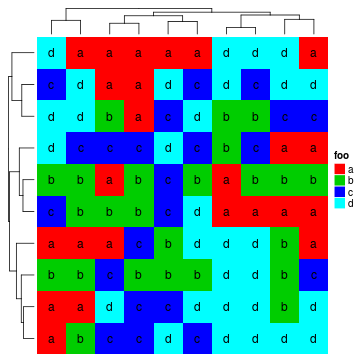Method to make hierarchical clustering can be specified by clustering_method_rows and clustering_method_columns. Possible methods are those supported in hclust() function.

Heatmap(mat, name = "foo", clustering_method_rows = "single")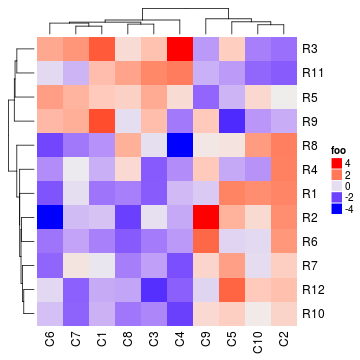By default, clustering is performed by hclust(). But you can also utilize clustering results which are generated by other methods by specifying cluster_rows or cluster_columns to a hclust or dendrogram object. In following examples, we use diana() and agnes() methods which are from the cluster package to perform clusterings.

library(cluster)
Heatmap(mat, name = "foo", cluster_rows = as.dendrogram(diana(mat)),
cluster_columns = as.dendrogram(agnes(t(mat))))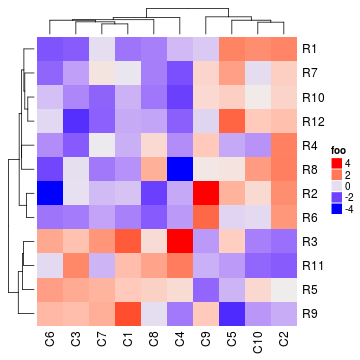In the native heatmap() function, dendrograms on row and on column are reordered to let features with larger different separated more from each other, By default the reordering for the dendrograms are turned on by Heatmap() as well.

Besides the default reordering method, you can first generate a dendrogram and apply other reordering method and then send the reordered dendrogram to cluster_rows argument.

Compare following three plots:

pushViewport(viewport(layout = grid.layout(nr = 1, nc = 3)))
pushViewport(viewport(layout.pos.row = 1, layout.pos.col = 1))
draw(Heatmap(mat, name = "foo", row_dend_reorder = FALSE, column_title = "no reordering"), newpage = FALSE)
upViewport()

pushViewport(viewport(layout.pos.row = 1, layout.pos.col = 2))
draw(Heatmap(mat, name = "foo", row_dend_reorder = TRUE, column_title = "applied reordering"), newpage = FALSE)
upViewport()

library(dendsort)
dend = dendsort(hclust(dist(mat)))
pushViewport(viewport(layout.pos.row = 1, layout.pos.col = 3))
draw(Heatmap(mat, name = "foo", cluster_rows = dend, row_dend_reorder = FALSE,
column_title = "reordering by dendsort"), newpage = FALSE)
upViewport(2)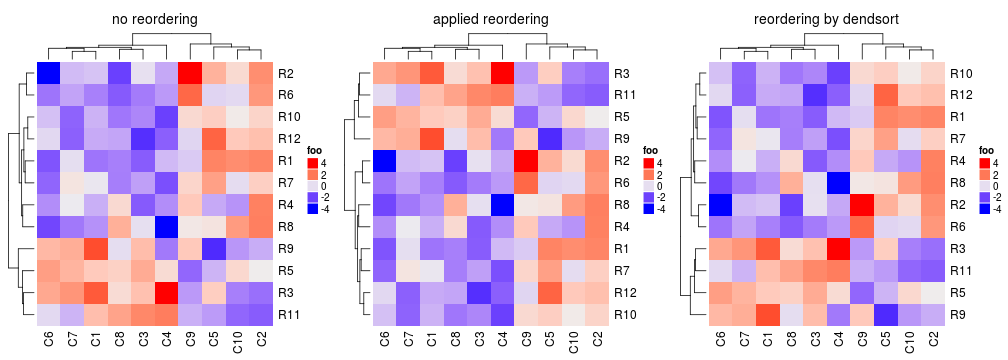You can render your dendrogram object by the dendextend package and make a more customized visualization of the dendrogram.

library(dendextend)
dend = hclust(dist(mat))
dend = color_branches(dend, k = 2)
Heatmap(mat, name = "foo", cluster_rows = dend)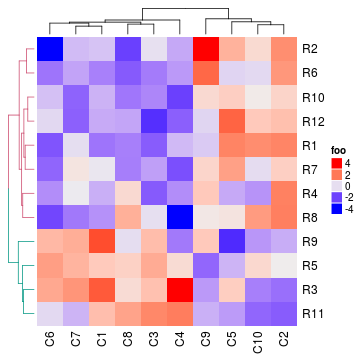More generally, cluster_rows and cluster_columns can be functions which calculate the clusterings. The input argument for the self-defined function should be a matrix and returned value should be a hclust or dendrogram object. Please note, when cluster_rows is executed internally, the argument m is the input mat itself while m is the transpose of mat when executing cluster_columns.

Heatmap(mat, name = "foo", cluster_rows = function(m) as.dendrogram(diana(m)),
cluster_columns = function(m) as.dendrogram(agnes(m)))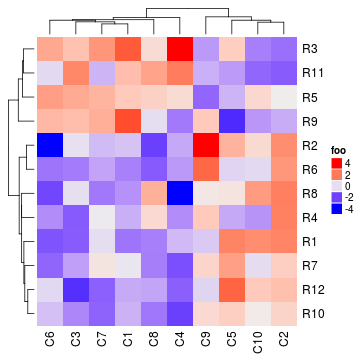fastcluster::hclust implements a faster version of hclust. We can re-define cluster_rows and cluster_columns to use the faster version of hclust. But note fastcluster::hclust only speed up the calculation of the cluster while not the calculation of distance matrix.

# code not run when building the vignette
Heatmap(mat, name = "foo", cluster_rows = function(m) fastcluster::hclust(dist(m)),
cluster_columns = function(m) fastcluster::hclust(dist(m))) # for column cluster, m will be automatically transposedTo make it more convinient to use the faster version of hclust (assuming you have many heatmaps to be concatenated), it can be set as a global option:

# code not run when building the vignette
ht_global_opt(fast_hclust = TRUE)
# now hclust from fastcluster package is used in all heatmaps
Heatmap(mat, name = "foo")Clustering can help to adjust order in rows and in columns. But you can still set the order manually by row_order and column_order. Note you need to turn off clustering if you want to set order manually. row_order and column_order can also be set according to matrix row names and column names if they exist.

Heatmap(mat, name = "foo", cluster_rows = FALSE, cluster_columns = FALSE,
row_order = 12:1, column_order = 10:1)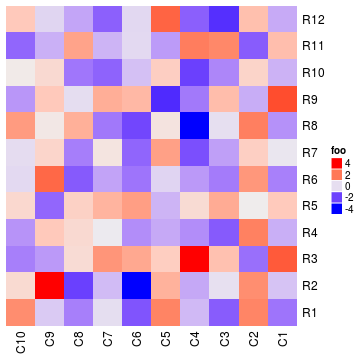Note row_dend_reorder and row_order are different. row_dend_reorder is applied on the dendrogram. Because for any node in the dendrogram, rotating two leaves gives an identical dendrogram. Thus, reordering the dendrogram by automatically rotating sub-dendrogram at every node will help to separate elements with more difference to be farther from each other. While row_order is applied on the matrix and dendrograms are suppressed.

## Dimension names

Side, visibility and graphic parameters for dimension names can be set as follows.

Heatmap(mat, name = "foo", row_names_side = "left", row_dend_side = "right",
column_names_side = "top", column_dend_side = "bottom")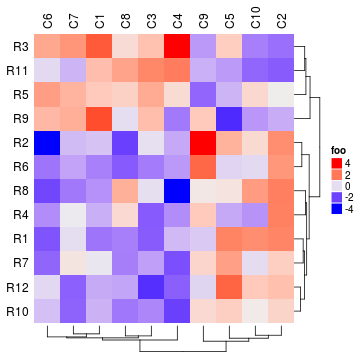Heatmap(mat, name = "foo", show_row_names = FALSE)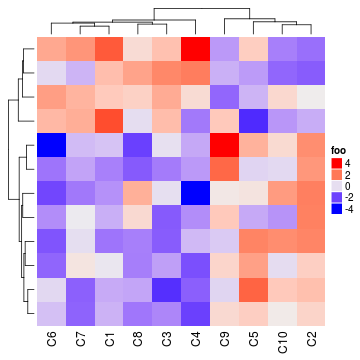Heatmap(mat, name = "foo", row_names_gp = gpar(fontsize = 20))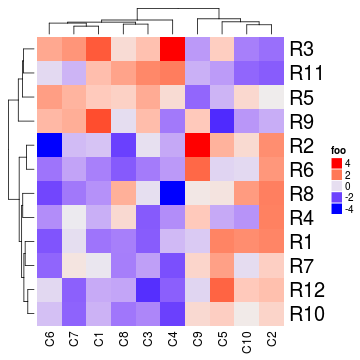Heatmap(mat, name = "foo", row_names_gp = gpar(col = c(rep("red", 4), rep("blue", 8))))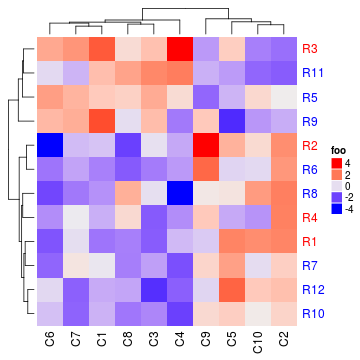Currently, rotations for column names and row names are not supported (or maybe in the future versions). Because after the text rotation, the dimension names will go inside other heatmap components which will mess up the heatmap layout. However, as will be introduced in Heatmap Annotation vignette, text rotation is allowed in the heatmap annotations. Thus, users can provide a row annotation or column annotation which only contains rotated text to simulate rotated row/column names (You will see the example in the Heatmap Annotation vignette).

## Split heatmap by rows

A heatmap can be split by rows. This will enhance the visualization of group separation in the heatmap. The km argument with a value larger than 1 means applying a k-means clustering on rows and clustering is applied on every k-means cluster.

Heatmap(mat, name = "foo", km = 2)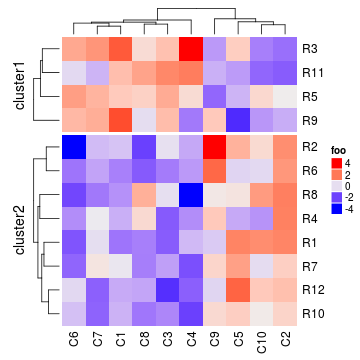More generally, split can be set to a vector or a data frame in which different combination of levels split the rows of the heatmap. Actually, k-means clustering just generates a vector of row classes and appends split with one additional column. The combined row titles for each row slice can be controlled by combined_name_fun argument. The order of each slice can be controlled by levels of each variable in split.

Heatmap(mat, name = "foo", split = rep(c("A", "B"), 6))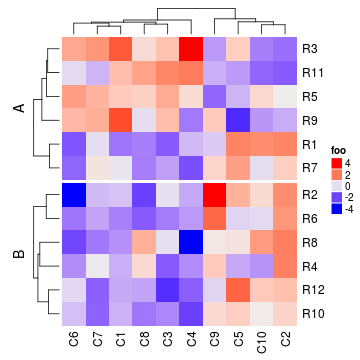Heatmap(mat, name = "foo", split = data.frame(rep(c("A", "B"), 6), rep(c("C", "D"), each = 6)))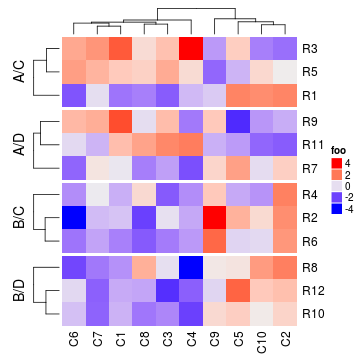Heatmap(mat, name = "foo", split = data.frame(rep(c("A", "B"), 6), rep(c("C", "D"), each = 6)),
combined_name_fun = function(x) paste(x, collapse = "\n"))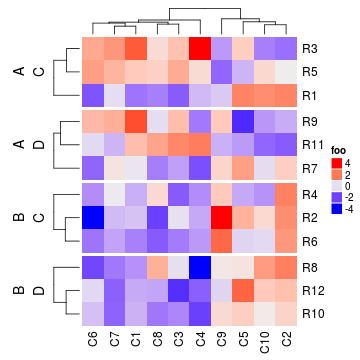Heatmap(mat, name = "foo", km = 2, split = factor(rep(c("A", "B"), 6), levels = c("B", "A")),
combined_name_fun = function(x) paste(x, collapse = "\n"))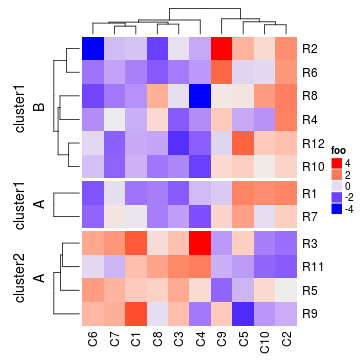Heatmap(mat, name = "foo", km = 2, split = rep(c("A", "B"), 6), combined_name_fun = NULL)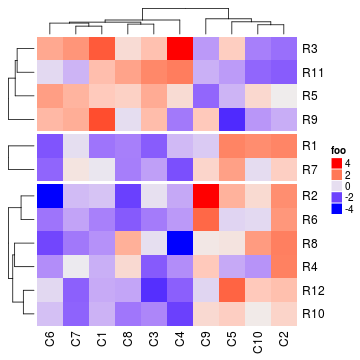If you are not happy with the default k-means partitioning method, it is easy to use other partitioning methods by just assigning the partitioning vector to split.

pa = pam(mat, k = 3)
Heatmap(mat, name = "foo", split = paste0("pam", pa$clustering))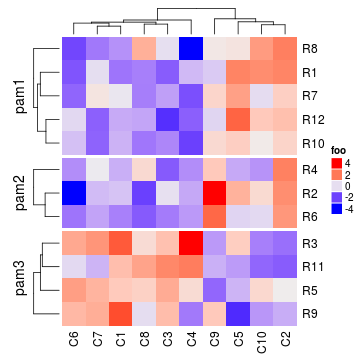If row_order is set, in each slice, rows are still ordered. Heatmap(mat, name = "foo", row_order = 12:1, cluster_rows = FALSE, km = 2)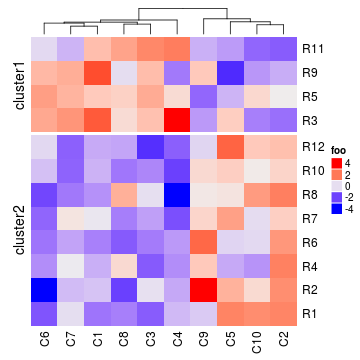Height of gaps between row slices can be controlled by gap (a single unit or a vector of units). Heatmap(mat, name = "foo", split = paste0("pam", pa$clustering), gap = unit(5, "mm"))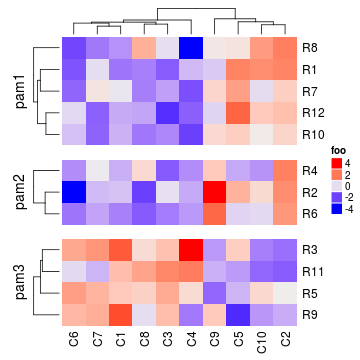Character matrix can only be split by split argument.

Heatmap(discrete_mat, name = "foo", col = 1:4,
split = rep(letters[1:2], each = 5))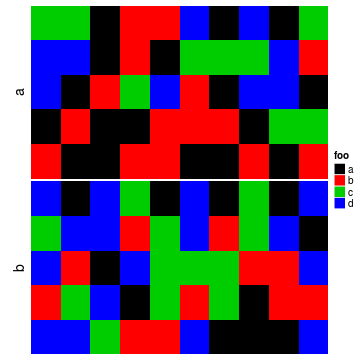When split is applied on rows, graphic parameters for row title and row names can be specified as same length as number of row slices.

Heatmap(mat, name = "foo", km = 2, row_title_gp = gpar(col = c("red", "blue"), font = 1:2),
row_names_gp = gpar(col = c("green", "orange"), fontsize = c(10, 14)))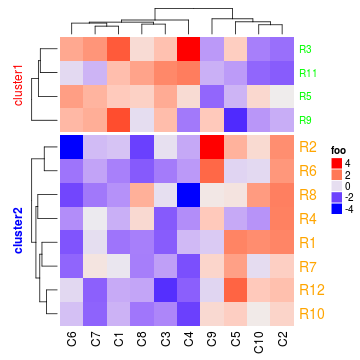Users may already have a dendrogram for rows and they want to split rows by splitting the dendrogram into k sub trees. In this case, split can be specified as a single number:

dend = hclust(dist(mat))
dend = color_branches(dend, k = 2)
Heatmap(mat, name = "foo", cluster_rows = dend, split = 2)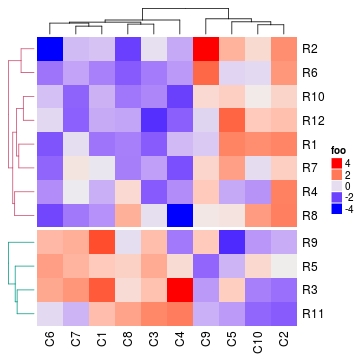Or they just split rows by specifying split as an integer. Note it is different from by km. If km is set, k-means clustering is applied first and clustering is applied to every k-mean cluster; while if split is an integer, clustering is applied to the whole matrix and later split by cutree().

Heatmap(mat, name = "foo", split = 2)